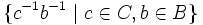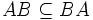# Lemma on inverse flower arrangement and product of subgroups

## History

This lemma appeared in Feit and Thompson's paper proving the odd-order theorem.

## Definition

Suppose$A, B, C$ are three subgroups of a group$G$ such that:$A \subseteq BC, \qquad B \subseteq CA, \qquad C \subseteq AB$

where$BC$ denotes the Product of subgroups (?)$B$ and$C$, then$AB = BA = BC = CB = CA = AC$, and in particular, the products$AB, BC, CA$ are subgroups. Thus,$A,B$ permute, as do$B,C$ and$C,A$.

## Proof

Given: Subgroups$A,B,C$ of a group$G$ such that$A \subseteq BC$,$B \subseteq CA$, and$C \subseteq AB$.

To prove:$AB = BA = BC = CB = CA = AC$.

Proof:

1.$(BC)^{-1} = CB$,$(CA)^{-1} = AC$ and$(AB)^{-1} = BA$: We first observe that$(BC)^{-1}$, i.e., the set of inverses of elements of$BC$, is given as the set$\{ c^{-1}b^{-1} \mid c \in C, b \in B \}$. Since the inverse map is bijective on a subgroup, we get$BC^{-1} = CB$.
2.$A \subseteq CB$,$B \subseteq AC$ and$C \subseteq BA$: Since$A \subseteq BC$,$A^{-1} \subseteq (BC)^{-1}$, yielding$A \subseteq CB$. Similar arguments show that$B \subseteq AC$ and$C \subseteq BA$.
3.$AB = BA$,$BC = CB$,$CA = AC$: We have$AB \subseteq (BC)(CA) = BCA \subseteq B(BA)A = BA$. Thus,$AB \subseteq BA$. Similarly,$BA \subseteq (AC)(CB) = ACB \subseteq A(AB)B = AB$. Thus,$AB = BA$.
4.$AB = BA = BC = CB = CA = AC$: We have$AB = BA \subseteq (CA)A = CA$. Similarly,$CA \subseteq BC$ and$BC \subseteq AB$. The chain of inclusions shows that all the members are equal.